# CBSE Term-2 Math(Basic) Class 10 Sample Question Paper 2022

Safalta expert Published by: Yashaswi More Updated Wed, 23 Mar 2022 10:03 PM IST

## Highlights

CBSE Sample Paper 2022 for Class 10 Maths (Basic) is available here, as well as the CBSE Marking Scheme. Examine the sample paper to become acquainted with the most recent paper pattern, and then prepare for your exam accordingly. Refer to the marking scheme for answers and solutions in a step-by-step fashion.

CBSE Term 2 Exam 2022: The Term 2 Sample Papers have been issued, allowing CBSE Class 10 students to begin efficient preparations for the forthcoming board exam. We have shared the CBSE Class 10 Maths (Basic) Sample Paper and its marking scheme in this article. This sample paper enables students to understand the pattern and weighting of questions asked in various areas of the examination, allowing them to prepare similar questions for the future board exam. The marking scheme can be used to determine the answers to all of the questions in the sample paper. It also aids in the understanding of how to write step-by-step solutions in a subjective-type exam in order to achieve high scores. Join Safalta School Online and prepare for Board Exams under the guidance of our expert faculty.

### Free Demo ClassesSource: Safalta.com

Our online school aims to help students prepare for Board Exams by ensuring that students have conceptual clarity in all the subjects and are able to score their maximum in the exams.

Also Check-
CBSE Class 10 Model Papers for Term 1 Exam : Download In PDF
CBSE results delayed for TERM-1 EXAMS 2021-22

### Check below CBSE Class 10 Mathematics (Basic) (241) Sample Paper Term 2 (2022):

Time Allowed: 2 hours

Maximum Marks: 40

General Instructions:

1.The question paper consists of 14 questions divided into 3 sections A, B, C.

2. All questions are compulsory.

3. Section A comprises of 6 questions of 2 marks each. Internal choice has been provided in two questions.

4. Section B comprises of 4 cbsequestions of 3 marks each. Internal choice has been provided in one question.

5. Section C comprises of 4 questions of 4 marks each. An internal choice has been provided in one question. It contains two case study based questions.

Section A

1. Find the roots of the quadratic equation 3x2−7x−6 = 0.

Or

Find the values of k for which the quadratic equation 3x2 + kx + 3 = 0  has real and equal roots.

2. Three cubes each of volume 64cm3 are joined end to end to form a cuboid. Find the total surface area of the cuboid so formed?

3. An inter house cricket match was organized by a school. Distribution of runs made by the students is given below. Find the median runs scored.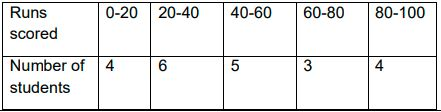4. Find the common difference of the AP 4, 9, 14,… If the first term changes to 6 and the common difference remains the same then write the new AP.

5.The mode of the following frequency distribution is 38. Find the value of x.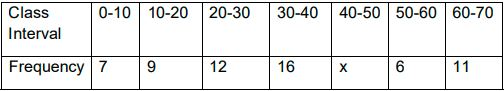6. XY and MN are the tangents drawn at the end points of the diameter DE of the circle with centre O. Prove that XY||MN.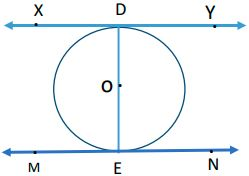OR

In the given figure, a circle is inscribed in the quadrilateral ABCD. Given AB=6cm, BC=7cm and CD=4cm. Find AD.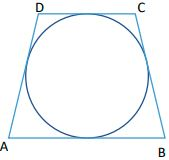Also check>>CBSE Term-2 Class 10 Social Science Syllabus 2022

Section-B

7. An AP 5, 8, 11…has 40 terms. Find the last term. Also find the sum of the last 10 terms.

8. A tree is broken due to the storm in such a way that the top of the tree touches the ground and makes an angle of 300 with the ground. Length of the broken upper part of the tree is 8 meters. Find the height of the tree before it was broken.

Or

Two poles of equal height are standing opposite each other on either side of the road 80m wide. From a point between them on the road the angles of elevation of the top of the two poles are respectively 600 and 300. Find the distance of the point from the two poles.

9. PA and PB are the tangents drawn to a circle with centre O. If PA= 6cm and ∠APB=600, then find the length of the chord AB.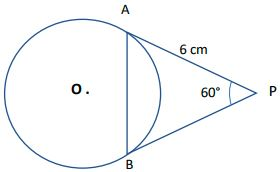10. The sum of the squares of three positive numbers that are consecutive multiples of 5 is 725. Find the three numbers.

Check>>>Class 10 CBSE Math Preparation Tips: Check These Tips To Ensure Full Marks
To check the preparation tips for scoring maximum marks in CBSE class 10 Social Studies Board Exam,

### What types of questions does section C comprises of ?

Section C comprises of 4 questions of 4 marks each. An internal choice has been provided in one question. It contains two case study based questions.

## Is there any internal choice in the question paper ?

There is no overall choice in the question paper .

## Is CBSE released sample paper for 2022 for Class 10?

CBSE Class 10 Sample Paper 2022 for the Term I exam has been released online in pdf form. The Central Board of Secondary Education has released the question banks separately for all subjects. The eligible students can also get to know the marking scheme. The sample papers are designed as per the revised syllabus

## Is board paper based on sample paper?

The Sample papers for classes 10th and class 12th are issued by CBSE atleast a month prior to the board exams. These sample papers are made by the panel of teachers at CBSE. The sample papers are issued so that the students can understand what to expect in the upcoming board exams.

## Is basic maths easy Class 10

Maths Basic Vs Maths Standard - Class 10. The Maths Basic Exam is easier than the Maths Standard Exam